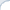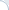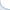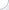This short post is an update for the previous blog entry. Most likely,
the Intel compiler does not produce the code that is 100x faster, at least, not under normal circumstances. The updated benchmark is posted on the GitHub. Note that the explanation below is just my guess, I will be happy to hear an alternative version.

It looks like the Intel compiler is super-clever and can dynamically adjust accuracy of computation. Consider the following code:

    float sum = 0;    for (int j = 0; j < rep; ++j) {        for (int i = 0; i < N*4; i+=4) {            sum += exp(data[i]);            sum += exp(data[i+1]);            sum += exp(data[i+2]);            sum += exp(data[i+3]);        }    }

Here the sum becomes huge very quickly. Thus, the result of calling exp becomes very small compared to sum. It appears to me that the code built with the Intel compiler does detect this situation. Probably, at run-time. After this happens, the function exp is computed using a very low-accuracy algorithm or not computed at all. As a result, when I ran this benchmark on a pre-historic Intel Core Duo 2GHz, I was still able to crunch billions of exp per second, which was clearly impossible. Consider now the following, updated, benchmark code:

    float sum = 0;    for (int j = 0; j < rep; ++j) {        for (int i = 0; i < N*4; i+=4) {            sum += exp(data[i]);            sum += exp(data[i+1]);            sum += exp(data[i+2]);            sum += exp(data[i+3]);        }       sum /= float(N*4); // Don't allow sum become huge!    }

Note line 9. It prevents sum from becoming huge. Now, we are getting more reasonable performance figures. In particular, for single-precision values, i.e., floats,
the Intel compiler produces a code that is only 10x faster compared to code produced by the GNU compiler. It is a large difference, but it is probably due to using SIMD
extensions for Intel.